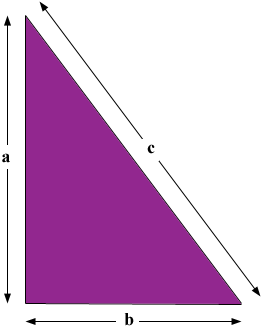SEARCH HOMEMath Central Quandaries & QueriesQuestion from Dannielle, a student: how do you find the hypotenuse if a=8 and b=6?Hi Dannielle,

You use Pythagoras theorem. The theorem says that if a right triangle has side lengths a, b and c where c is the length of the hypotenuse (the side opposite the right angle) then a2 + b2 = c2.For example suppose you have sides of length a = 3 inches and b = 4 inches then by Pythagoras theorem a2 + b2 = c2 so 32 + 42 = c2 and thus c2 = 9 + 16 = 25 and hence c = √25 = 5 inches.